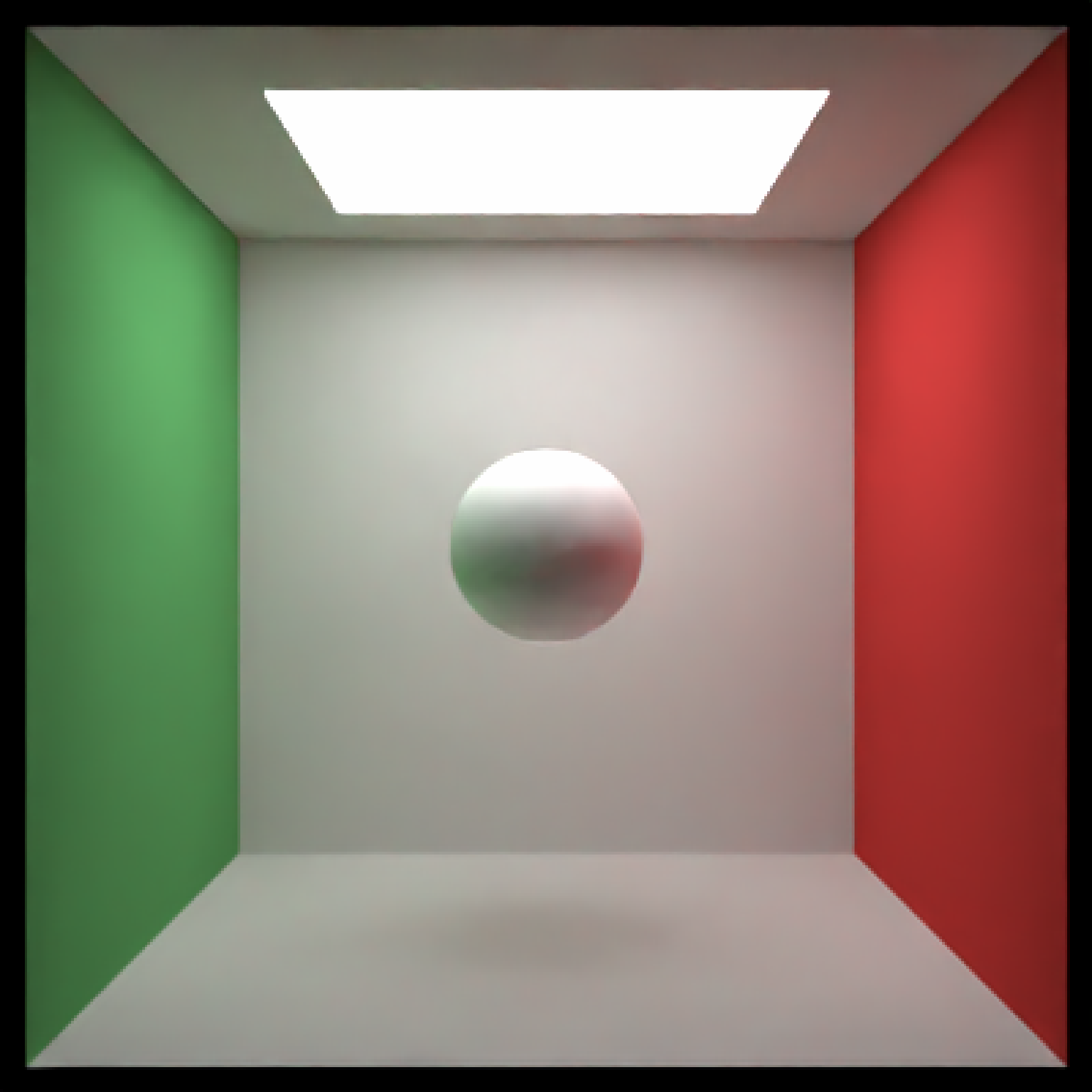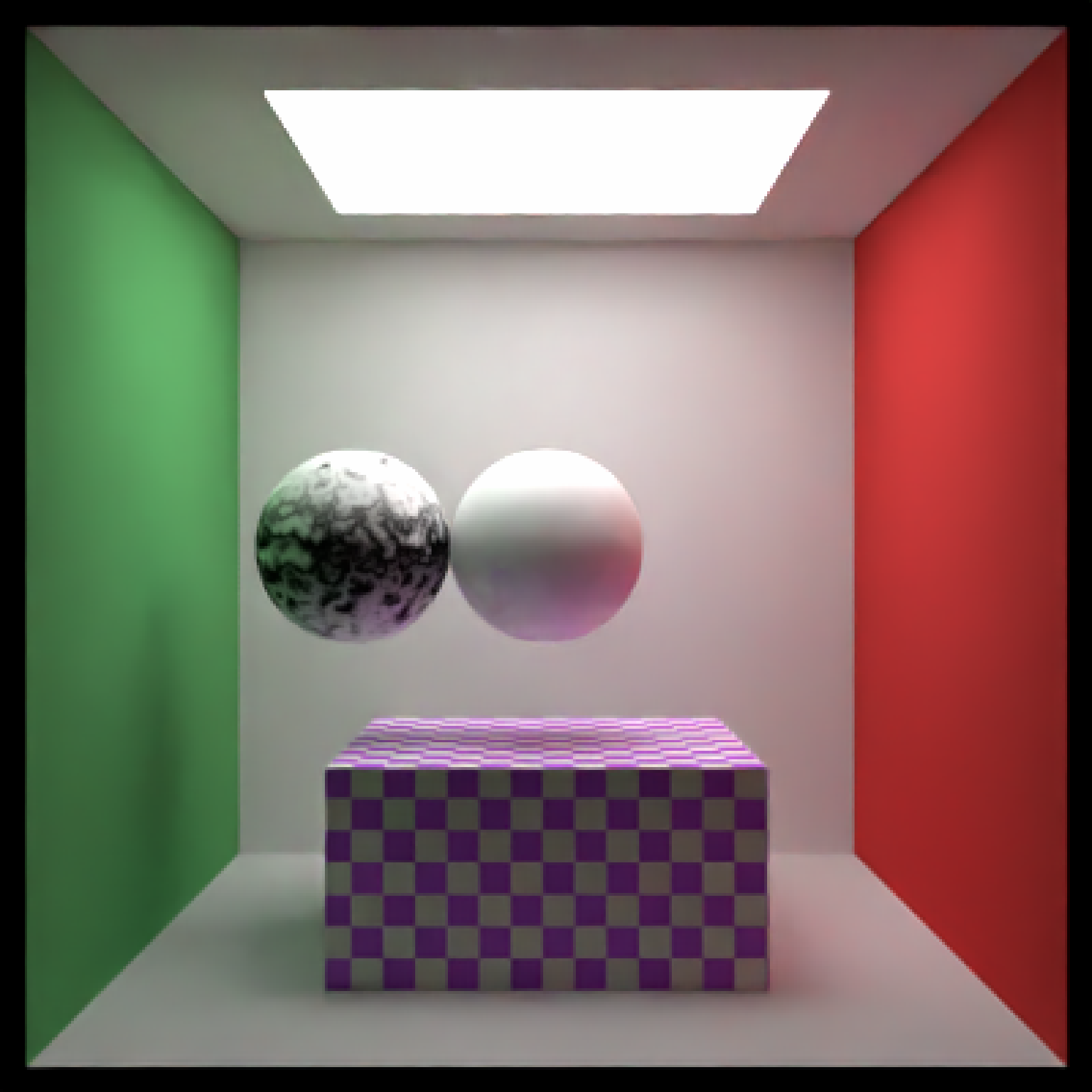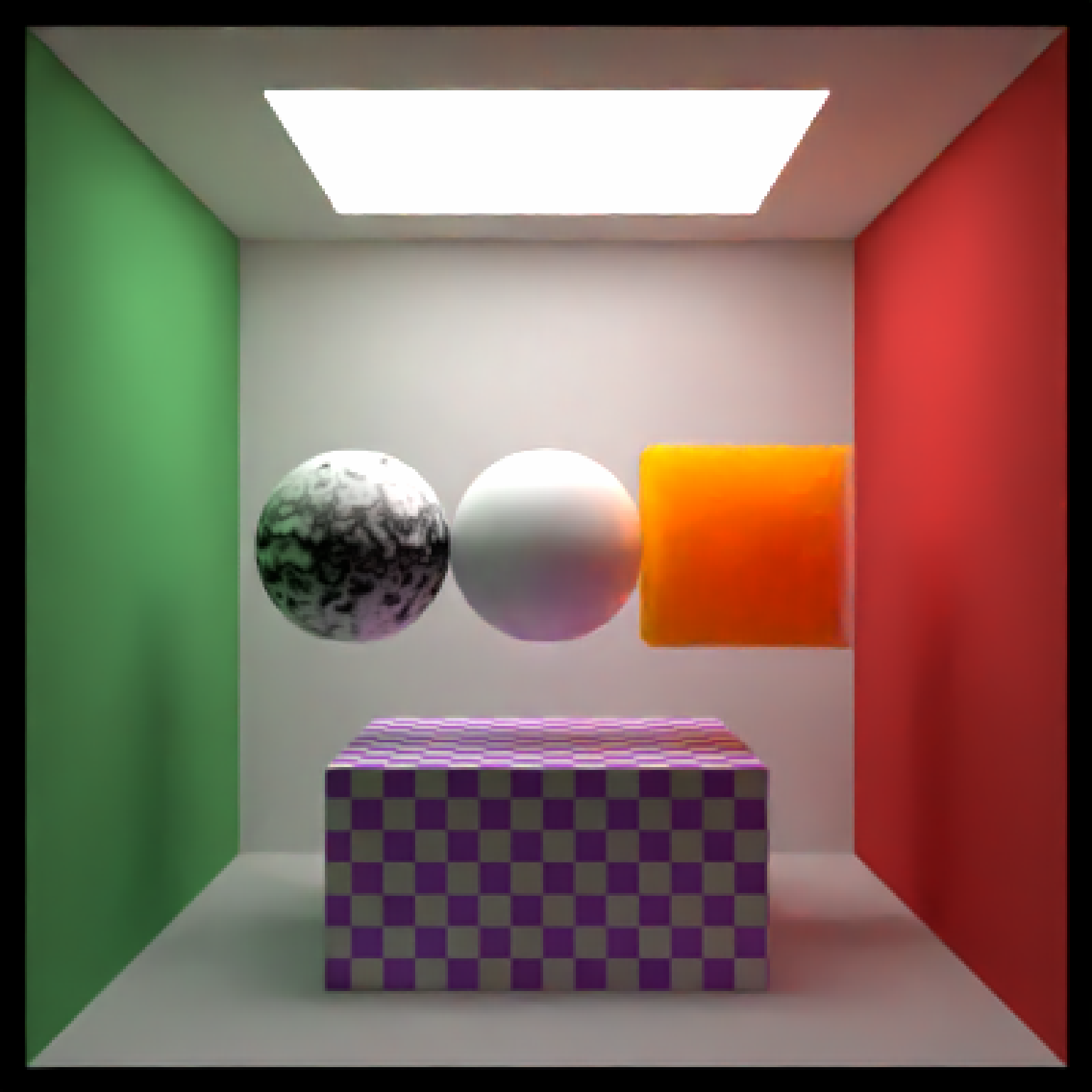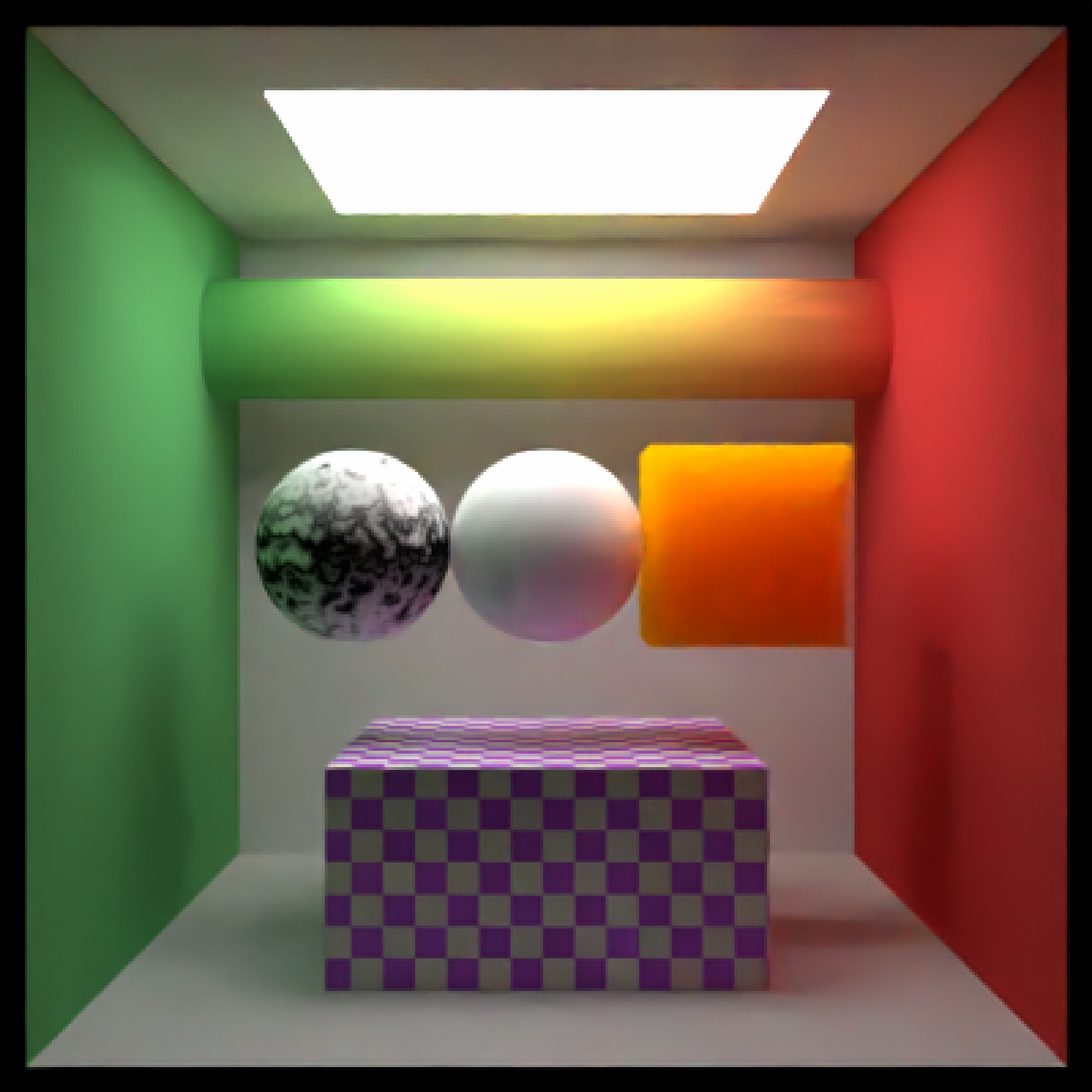Diffuse Material

diffuse(
color = "#ffffff",
checkercolor = NA,
checkerperiod = 3,
noise = 0,
noisephase = 0,
noiseintensity = 10,
noisecolor = "#000000",
image_texture = NA,
image_repeat = 1,
alpha_texture = NA,
bump_texture = NA,
bump_intensity = 1,
fog = FALSE,
fogdensity = 0.01,
sigma = NULL,
importance_sample = FALSE
)

## Arguments

color

Default white. The color of the surface. Can be either a hexadecimal code, R color string, or a numeric rgb vector listing three intensities between 0 and 1.

checkercolor

Default NA. If not NA, determines the secondary color of the checkered surface. Can be either a hexadecimal code, or a numeric rgb vector listing three intensities between 0 and 1.

checkerperiod

Default 3. The period of the checker pattern. Increasing this value makes the checker pattern bigger, and decreasing it makes it smaller

noise

Default 0. If not 0, covers the surface in a turbulent marble pattern. This value will determine the amount of turbulence in the texture.

noisephase

Default 0. The phase of the noise. The noise will repeat at 360.

noiseintensity

Default 10. Intensity of the noise.

noisecolor

Default #000000. The secondary color of the noise pattern. Can be either a hexadecimal code, or a numeric rgb vector listing three intensities between 0 and 1.

Default NA. If not NA, creates a secondary color for a linear gradient between the this color and color specified in color. Direction is determined by gradient_transpose.

Default FALSE. If TRUE, this will use the v coordinate texture instead of the u coordinate texture to map the gradient.

Default NA. If not NA, this changes the behavior from mapping texture coordinates to mapping to world space coordinates. This should be a length-3 vector specifying the x,y, and z points where the gradient begins with value color.

Default NA. If not NA, this changes the behavior from mapping texture coordinates to mapping to world space coordinates. This should be a length-3 vector specifying the x,y, and z points where the gradient begins with value gradient_color.

Default hsv. Colorspace to calculate the gradient. Alternative rgb.

image_texture

Default NA. A 3-layer RGB array or filename to be used as the texture on the surface of the object.

image_repeat

Default 1. Number of times to repeat the image across the surface. u and v repeat amount can be set independently if user passes in a length-2 vector.

alpha_texture

Default NA. A matrix or filename (specifying a greyscale image) to be used to specify the transparency.

bump_texture

Default NA. A matrix, array, or filename (specifying a greyscale image) to be used to specify a bump map for the surface.

bump_intensity

Default 1. Intensity of the bump map. High values may lead to unphysical results.

fog

Default FALSE. If TRUE, the object will be a volumetric scatterer.

fogdensity

Default 0.01. The density of the fog. Higher values will produce more opaque objects.

sigma

Default NULL. A number between 0 and Infinity specifying the roughness of the surface using the Oren-Nayar microfacet model. Higher numbers indicate a roughed surface, where sigma is the standard deviation of the microfacet orientation angle. When 0, this reverts to the default lambertian behavior.

importance_sample

Default FALSE. If TRUE, the object will be sampled explicitly during the rendering process. If the object is particularly important in contributing to the light paths in the image (e.g. light sources, refracting glass ball with caustics, metal objects concentrating light), this will help with the convergence of the image.

## Value

Single row of a tibble describing the diffuse material.

## Examples

#Generate the cornell box and add a single white sphere to the center
scene = generate_cornell() %>%
if(run_documentation()) {
render_scene(scene, lookfrom=c(278,278,-800),lookat = c(278,278,0), samples=128,
aperture=0, fov=40, ambient_light=FALSE, parallel=TRUE)
}#Add a checkered rectangular cube below
scene = scene %>%
material = diffuse(checkercolor="purple",checkerperiod=20)))
if(run_documentation()) {
render_scene(scene, lookfrom=c(278,278,-800),lookat = c(278,278,0), samples=128,
aperture=0, fov=40, ambient_light=FALSE, parallel=TRUE)
}scene = scene %>%
material = diffuse(noise=1/20)))
if(run_documentation()) {
render_scene(scene, lookfrom=c(278,278,-800),lookat = c(278,278,0), samples=128,
aperture=0, fov=40, ambient_light=FALSE, parallel=TRUE)
}#Add an orange volumetric (fog) cube
scene = scene %>%
material = diffuse(fog=TRUE, fogdensity=0.05,color="orange")))
if(run_documentation()) {
render_scene(scene, lookfrom=c(278,278,-800),lookat = c(278,278,0), samples=128,
aperture=0, fov=40, ambient_light=FALSE, parallel=TRUE)
}